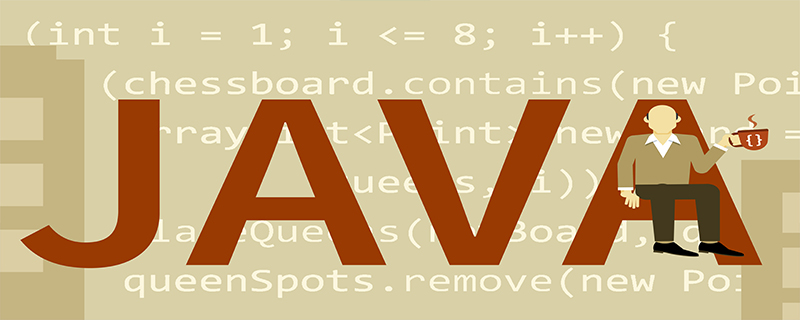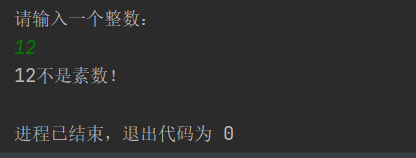• 判断一个数是否质数(素数)方法：如果是偶数，直接返回；然后从3开始，步长为2，一直到n的算术平方根为止，都除不尽则为质数Java程序：(推荐：java视频教程)public class Main {public static void main(String[]...质数的定义：
对于大于1的数，如果除了1和它本身，它不能再被其它正整数整除，那么我们说它是一个质数。
判断一个数是否为质数(素数)方法：
如果是偶数，直接返回；然后从3开始，步长为2，一直到n的算术平方根为止，都除不尽则为质数。
Java程序：(推荐：java视频教程)public class Main {public static void main(String[] args) {for (int j = 0; j <= 19; j++) {System.out.println(j+": "+isPrime(j) );}}private static boolean isPrime(int src) {double sqrt = Math.sqrt(src);if (src < 2) {return false;}if (src == 2 || src == 3) {return true;}if (src % 2 == 0) {// 先判断是否为偶数，若偶数就直接结束程序return false;}for (int i = 3; i <= sqrt; i+=2) {if (src % i == 0) {return false;}}return true;}}
更多java知识请关注java基础教程栏目。

展开全文• Java键盘输入一个数n，判断质数素数）方法 import java.util.Scanner; public class test{ public static void main(String[] args){ System.out.print("请输入一个大于1的数判断是否质数:"); Scanner in=new...
题目1:判断101-200之间有多少个（质数）素数，并输出所有素数。
/**
*	判断素数的方法：用一个数分别去除2到sqrt(这个数)，
*	如果能被整除，则表明此数不是素数，反之是素数。
*/

// 方法一 遍历范围数
public class Demo {
public static void main(String[] args) {
// 2,3为素数所以计数初始值为2
int count = 2;
// 从2到sqrt(i)找出整除数，所以从i = 4开始
for (int i = 4; i <= 200; i++) {
for (int j = 2; j <= Math.sqrt(i); j++) {
// 先遍历2到sqrt(i)中有没有可以被整除的，若有则为素数退出当前循环
if (i % j == 0) {
System.out.println(i + "这个数不是素数!");
break;
}
// i % j != 0判定完毕，但不清楚是否遍历到sqrt(i)
// 所以加一层判定sqrt(i) - j < 1输出素数i;
//
else if (Math.sqrt(i)-j < 1) {
++count;
System.out.println(i + "这个数是素数!");
}
}
}
System.out.println("共有" + count + "个素数");
}
}

// 方法二 键盘输入数据进行判断,创建判断质数方法
import java.util.Scanner;
public class Demo {
public static void main(String[] args) {
System.out.print("请输入一个大于1的数判断是否为质数:");
Scanner in = new Scanner(System.in);
int n = in.nextInt();
if(isPrime(n)) {
System.out.println(n+ "是质数！");
}
else {
System.out.println(n+ "不是质数！");
}
}
public static boolean isPrime(int n) {
boolean flag = true;
// Math.sqrt(n),n的平方根
// 质数判断,在[2,Math.sqrt(n)]范围内,没有一个数能整除n,则n是质数
for(int i = 2;i <= Math.sqrt(n); i++) {
if(n%i == 0) {
flag = false;
break;
}
}
return flag;
}
}

// 方法三 在遍历数时创建int flag(进行01判断)
public class Demo {
public static void main(String[] args) {
int count = 2;
for (int i = 4; i <= 200; i++) {
int flag = 0;
for (int j = 2; j <= Math.sqrt(i); j++) {
if (i % j == 0) {
System.out.println(i + "不是素数!");
// 如果说数第一次取余等于0，则不为素数，count=1
flag = 1;
break;
}
}
// 从2到sqrt(i)遍历完成，如果count<1则为素数
if (flag < 1) {
++count;
System.out.println(i + "是素数!");
}
}
System.out.println("一共有" + count + "个素数！");
}
}

展开全文• 判断是否质数，最优化的运行效率，减少性能损耗。测试计算机的运算速度。java学习的入门基础，最优化计算时间
• java判断一个整数是不是素数质数） /** * 判断一个数是不是素数质数） * 质数是指在大于1的自然数中，除了1和它本身以外不再有其他因数的自然数。 */ import java.util.Scanner; public class Test09 { ...
java判断一个整数是不是素数（质数）
/**
* 判断一个数是不是素数（质数）
* 质数是指在大于1的自然数中，除了1和它本身以外不再有其他因数的自然数。
*/

import java.util.Scanner;

public class Test09 {
public static void main(String[] args) {
Scanner sc = new Scanner(System.in);
System.out.println("请输入一个整数：");
int num = sc.nextInt();
int i = 2;
boolean flag = true;
while (i < num) {       //不要在循环中做输出，在循环中做标记
if (num % i == 0) {
flag = false;
}
i++;
}
if (flag == true) {
System.out.println(num+"是素数！");
} else {
System.out.println(num+"不是素数！");
}
sc.close();
}
}

执行代码结果如下：素数（质数）的概念;
百度百科直达车：https://baike.baidu.com/item/%E8%B4%A8%E6%95%B0/263515?fromtitle=%E7%B4%A0%E6%95%B0&fromid=115069
展开全文jvm 字符串 leetcode js
• 　如果一个正整数只有两个因子, 1和p，则称p为素数. 　public boolean isPrime(int n) 　{ 　if(n 　for(int i = 2; i 　if(n%i == 0) return false; 　return true; 　} 　时间复杂度O(n). 　2. 改进, ...
　1. 根据概念判断:
如果一个正整数只有两个因子, 1和p，则称p为素数.
public boolean isPrime(int n)
{
if(n < 2) return false;
for(int i = 2; i < n; ++i)
if(n%i == 0) return false;
return true;
}
时间复杂度O(n).
2. 改进, 去掉偶数的判断
public boolean isPrime(int n)
{
if(n < 2) return false;
if(n == 2) return true;
if(n%2==0) return false;
for(int i = 3; i < n; i += 2)
if(n%i == 0) return false;
return true;
}
时间复杂度O(n/2), 速度提高一倍.
3. 进一步减少判断的范围
定理: 如果n不是素数, 则n有满足1< d<=sqrt(n)的一个因子d.
证明: 如果n不是素数, 则由定义n有一个因子d满足1< d< n.
如果d大于sqrt(n), 则n/d是满足1< n/d<=sqrt(n)的一个因子.
public boolean isPrime(int n)
{
if(n < 2) return false;
if(n == 2) return true;
if(n%2==0) return false;
for(int i = 3; i*i <= n; i += 2)
if(n%i == 0) return false;
return true;
}
时间复杂度O(Math.sqrt(n)/2), 速度提高O((n-Math.sqrt(n))/2).
4. 剔除因子中的重复判断.
定理: 如果n不是素数, 则n有满足1< d<=Math.sqrt(n)的一个"素数"因子d.
证明: I1. 如果n不是素数, 则n有满足1< d<=Math.sqrt(n)的一个因子d.
I2. 如果d是素数, 则定理得证, 算法终止.
I3. 令n=d, 并转到步骤I1.
由于不可能无限分解n的因子, 因此上述证明的算法最终会停止.
// primes是递增的素数序列: 2, 3, 5, 7, ...
// 更准确地说primes序列包含1->Math.sqrt(n)范围内的所有素数
public boolean isPrime(int primes[], int n)
{
if(n < 2) return false;
for(int i = 0; primes[i]*primes[i] <= n; ++i)
if(n%primes[i] == 0) return false;
return true;
}
5. 构造素数序列primes: 2, 3, 5, 7, ...
由4的算法我们知道, 在素数序列已经被构造的情况下, 判断n是否为素数效率很高;
下面程序可以输出素数表.
int sqrtRoot;
int cursor = 0;
boolean isPrime;
for(int i=2;i<=maxNum;i++){
sqrtRoot = (int)Math.sqrt(i); //取平方根
isPrime = true;
for(int j=0;j< cursor;j++){
break;
isPrime = false;
break;
}
}
if(isPrime){
}
}
int[] result = new int[cursor];
return result;
}
public static void main(String[] args) throws Exception{
int maxNum = Integer.parseInt(args);
}
}
}
6.(在素数表中)二分查找
Arrays.BinarySearch方法:
该方法用于在指定数组中查找给定的值，采用二分法实现，所以要求传入的数组已经是排序了的。
该方法的基本语法格式为：
Static int binarySearch(byte[] a, byte key)
该方法返回数据中key元素所在的位置，如果没有key元素，则返回key应插入的位置：-（insertionpoint-1），如数组中的第一个元素就大于key，返回-1。
注：数组的数据类型可以是int[] byte[] short[] float[] long[] double[]char[] Object[]类型。
展开全文• ## JAVA判断是否为质数

千次阅读 2020-02-16 23:12:56
法一(for循环)： 思路：对2——num/2的数遍历，如果num除以2——num/2之间的数有余数的话，就说明num为质数。 下面通过代码实现： import java.util.Scanner;... // 输入一个数并判断是否质数 System.out.p...算法
• 质数(又称素数),是指在大于1的...//判断是否素数，1不是素数。 public static boolean judge(int x){ for(int i=2;i<x;i++){//在2 到 x-1 之间有数可以整除，不是素数。 if(x%i ==0){ return false; } }
• ## Java判断素数

千次阅读 2019-04-13 13:20:04
Java判断素数 public static void main(String[] args) { Scanner input=new Scanner(System.in); System.out.println("输入一个整数："); int n=input.nextInt(); if (isPrime(n)) { //方法 ...
• 判断质数 什么是质数 质数是指在大于1的自然数中，除了1和它本身以外不再有其他因数的自然数。 所以1不是质数 代码示例 public static boolean isPrime (int x) { if (x==1) { //1不是质数 return false; ...算法
• 素数质数） 定义 素数质数）是指在大于1的自然数中，除了1和它本身以外不再有其他因数的自然数。 —————————————— ...判断5是否素数 // An highlighted block int i=5; boolean prime=tru
• private static boolean isPrime(int a){ int k = (int) Math.sqrt(a);//k为num的正平方根，取整数 for (int i = 2; i <= k; i++) { if (a % i == 0) { ...//不是素数 } } return true ; }`算法
• 素数判断 java 2010-06-03 22:22 import java.io.*;import java.util.*;import java.math.*;public class Main {static Scanner in;static PrintWriter out;public static void main(String[] args) throws Ex...
• import java.util.*; class Prime{//注意无括号() private int m=0; public Prime(int m) { ... //判断是否素数:除了1和它本身外,不能被其他自然数整除(除0以外)的数为素数,否则为合数 public S...算法
• 判断回文数方法： 1、将数字倒转，进行比较。 static int reversal(int num) { //反转原数 int result = 0; while(num != 0){ int lastDigit = num % 10; result = result * 10+ lastDigit; ...
• ## JAVA判断素数

千次阅读 2018-03-22 22:52:23
问题 输入为一个数字n，要求输出比n小的素数对；素数对的意思是两个数为素数且它们之间的差为2，如3和5都是素数，且它们之间的差是2 // public class HelloWorld { static int flag;...判断是否素数 ...
• ## java判断一个数是否为素数/质数

万次阅读 多人点赞 2019-04-08 19:13:03
质数(prime number)又称素数，定义为一个大于1的自然数，除了1和它本身外，不能被其他自然数整除，换句话说就是该数除了1和它本身以外不再有其他的因数。 第一个思路 假如我们需要判断数字是33，因为所有的数都...c语言
• 质数又称为素数,定义如下: 质数。一个大于1的正整数，如果除了1和它本身以外，不能被其他正整数整除，就叫素数。如2，3，5，7，11，13，17…。 代码实例： import java.util.Scanner; public class Main{ public ......

# java判断是不是素数java 订阅